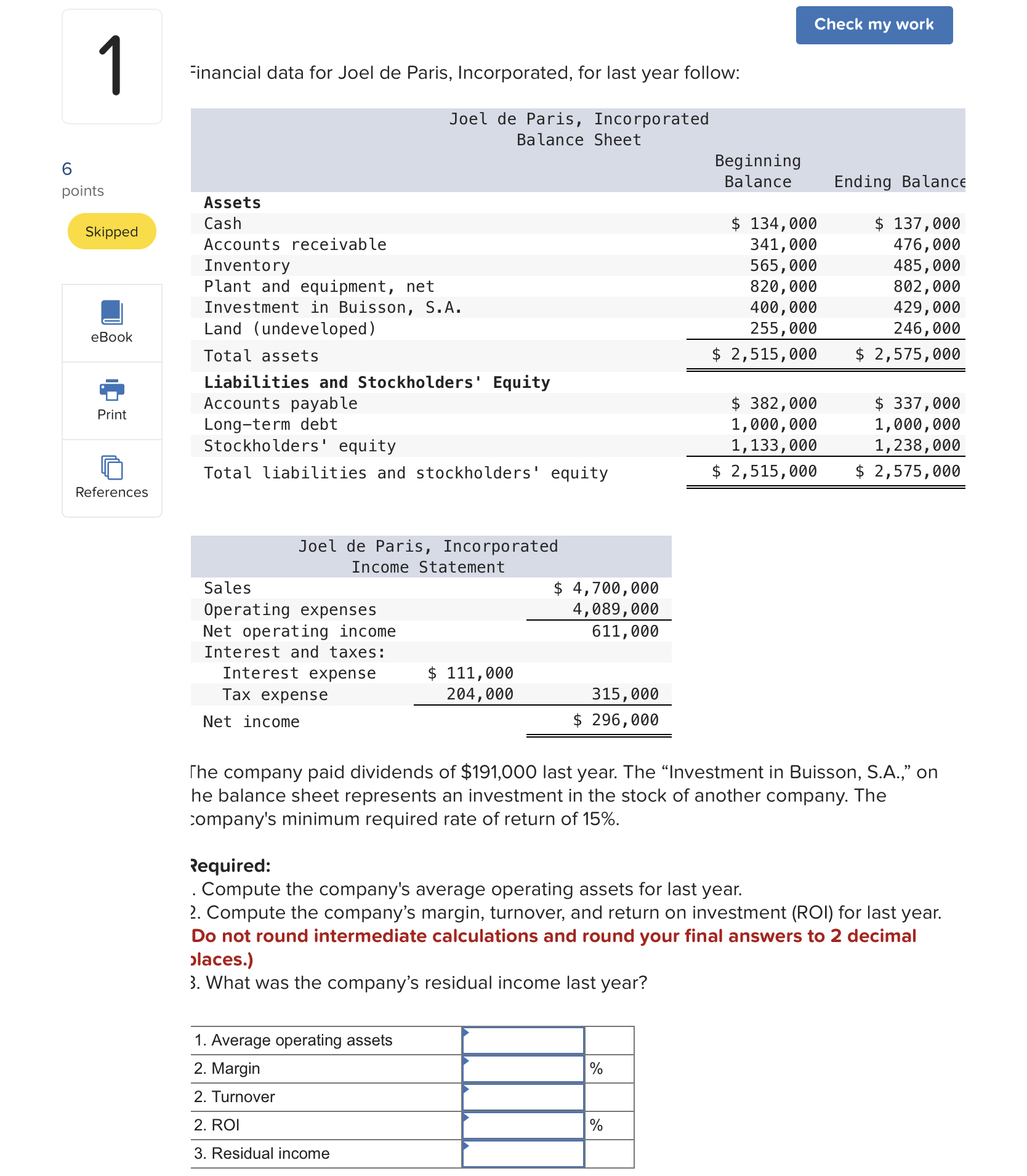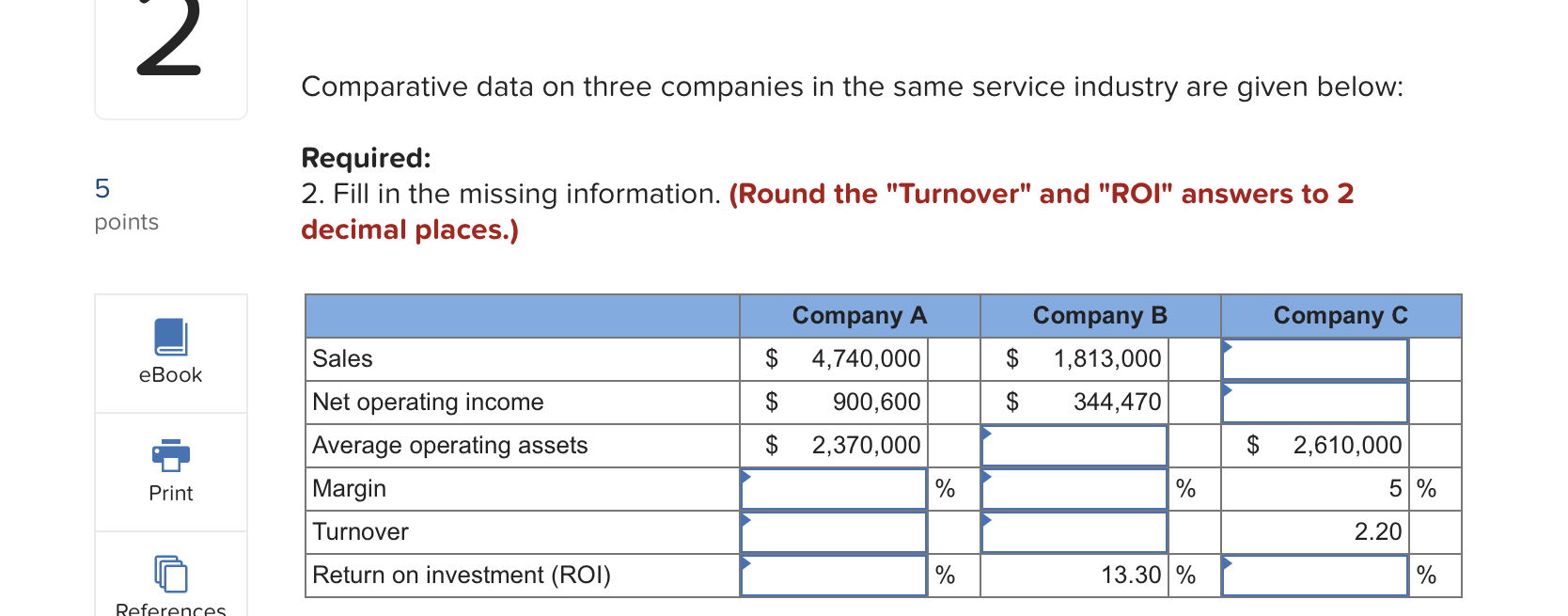Home / Expert Answers / Accounting / inancial-data-for-joel-de-paris-incorporated-for-last-year-follow-the-company-paid-dividends-of-pa116

# (Solved): -inancial data for Joel de Paris, Incorporated, for last year follow: The company paid dividends of ...-inancial data for Joel de Paris, Incorporated, for last year follow: The company paid dividends of last year. The "Investment in Buisson, S.A.," on he balance sheet represents an investment in the stock of another company. The :ompany's minimum required rate of return of . Pequired: . Compute the company's average operating assets for last year. 2. Compute the company's margin, turnover, and return on investment (ROI) for last year. Do not round intermediate calculations and round your final answers to 2 decimal slaces.) 3. What was the company's residual income last year? Comparative data on three companies in the same service industry are given below: Required: 2. Fill in the missing information. (Round the "Turnover" and "ROI" answers to 2 decimal places.)

We have an Answer from Expert

Average Operating Assets = (Beginning Balances + Ending Balances )/2
Average Operating Assets = ($1,860,000 +$1,900,000 )/2
Average Operating Assets = $1,880,000 Required 2 : Compute the Company's Margin , Turnover and Return on Investment (ROI) for Last Year : Margin = Net Operating Income / Sales Net Operating Income =$611,000
Sales = $4,700,000 Margin =$611,000 /$4,700,000 Margin = 13% Turnover = Sales / Average Opearting Assets Sales =$4,700,000
Average Opearting Assets  = $1,880,000 Turnover =$4,700,000 /$1,880,000 Turnover = 2.5 Return on Investment (ROI) = Margin* Turnover Margin = 13% Turnover = 2.5 Return on Investment (ROI) = Margin* Turnover Return on Investment (ROI) = 13%*2.5 Return on Investment (ROI) = 32.5% Calculate the Company's Residual Income Last Year : Minimum Required Return = Minimum Required rate of return *Average Operating Assets Minimum Required Return = 15% *$1,880,000
Minimum Required Return = $282,000 Net Operating Income =$611,000
Residual Income = Net Operating Income - Minimum Required Return
Residual Income = $611,000-$282,000
Residual Income = \$329,000
We have an Answer from Expert